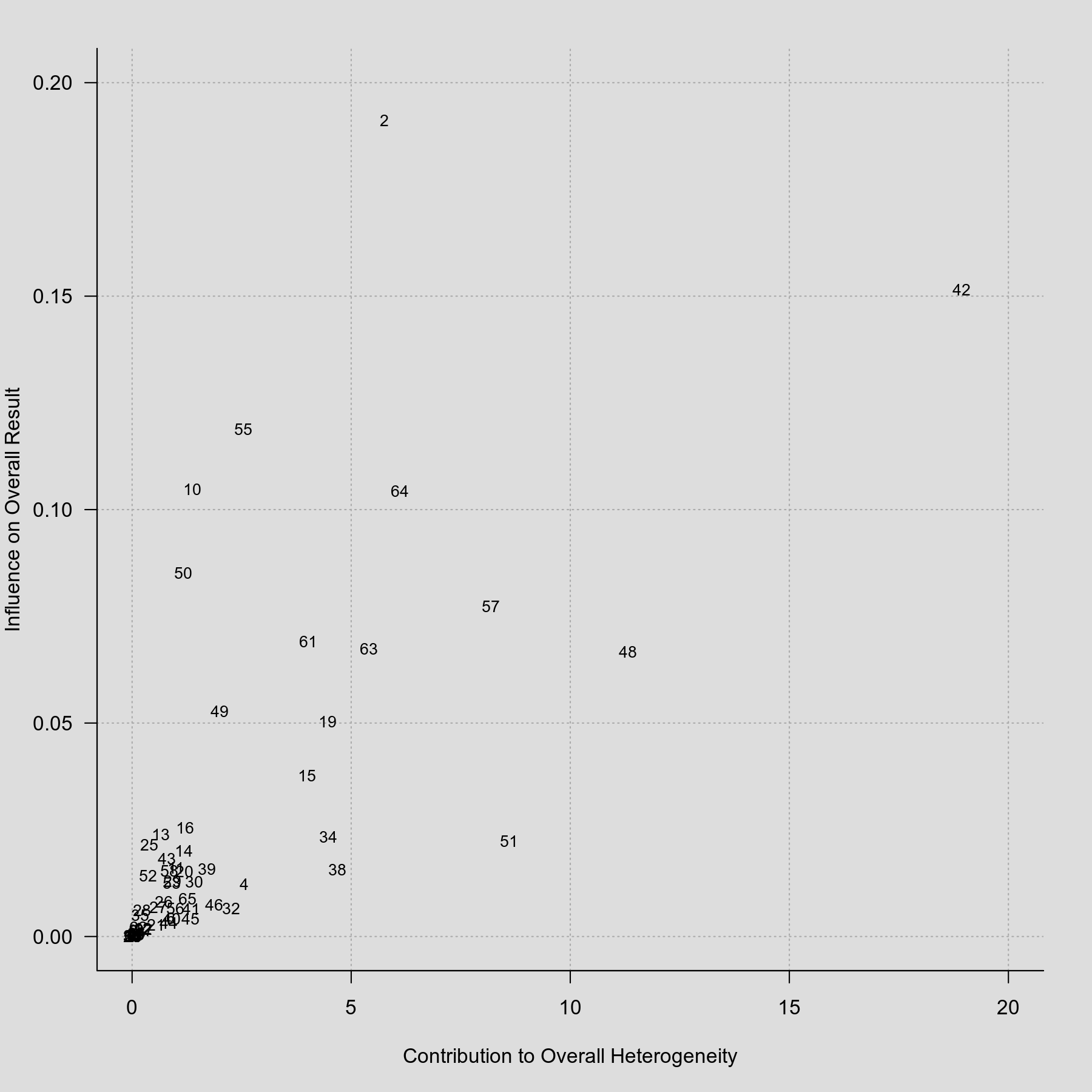#The metafor Package

A Meta-Analysis Package for R

### Sidebar

Action disabled: recent
plots:baujat_plot

## Baujat Plot

### Description

Baujat et al. (2002) proposed a diagnostic plot to detect sources of heterogeneity in meta-analytic data. The plot shows the contribution of each study to the overall Q-test statistic for heterogeneity on the horizontal axis versus the influence of each study (defined as the standardized squared difference between the overall estimate based on an equal-effects model with and without the $i$th study included in the model) on the vertical axis. An example of such a plot is shown below.

### Plot### Code

library(metafor)

### get data from Pignon et al. (2000)
dat <- dat.pignon2000

### compute estimated log hazard ratios and sampling variances
dat$yi <- with(dat, OmE/V) dat$vi <- with(dat, 1/V)

### meta-analysis based on all 65 trials
res <- rma(yi, vi, data=dat, method="EE", slab=id)

### create Baujat plot
baujat(res, xlim=c(0,20), ylim=c(0,0.2))

Baujat, B., Mahé, C., Pignon, J.-P., & Hill, C. (2002). A graphical method for exploring heterogeneity in meta-analyses: Application to a meta-analysis of 65 trials. Statistics in Medicine, 21(18), 2641–2652.

Pignon, J. P., Bourhis, J., Domenge, C., & Designe, L. (2000). Chemotherapy added to locoregional treatment for head and neck squamous-cell carcinoma: Three meta-analyses of updated individual data. Lancet, 355(9208), 949–955.

### Page Tools